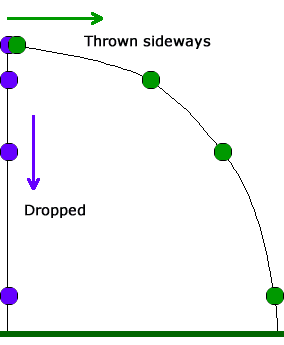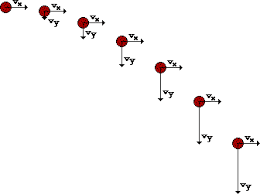# Velocity Formulas for Projectile Motion

• chromechris1
In summary, the issue Chromechris is experiencing is that she is not able to calculate the velocity using the d/t formula for constant velocity, but is able to using the v=gt equation for velocity as a result of acceleration.f

#### chromechris1

Homework Statement
A person stands on top of a 45-m cliff and throws a 500-gram rock forward with a speed of 40 m/sec. What is the speed of the rock when it hits the ground. Solve using energy and using kinematic equations.
Relevant Equations
kinematic equations
Hi, so I solved this using energy. I also tried solving this using kinematic equations. The issue I am running into s for calculating the Vy of the problem. I am able to find the time it takes the rock to fall using d=(1/2)gt^2. Then, I considered two different ways of going about finding the Vy. I can simply: Vy=gt. But then I also remembered that V=(d/t). So then I tried V=45/3 (I am using g=10m/s^2). The first formula yields the correct answer, but the second does not. Why is it that I cannot use the second formula if it is true that V=(d/t). My guess would be that it is because in the formula V=(d/t), we are considering calculating a constant velocity but in the second formula we are calculating velocity as a result of acceleration? Thank you for any insight into this.

Hi @chromechris1 and welcome to PF.

You cannot use V=d/t because there is acceleration in the vertical direction. Have you considered using the kinematic equation ##2ay=v_y^2-v_{0y}^2?##

•chromechris1
Thank you! I see. So does that also mean that I would not be able to use V=d/t for horizontal velocity if there is acceleration involved as well?

The reason we can't use V=d/t directly is that the problem is a Projectile Motion Problem. In projectile motion we simplify the motion into 2 components (x,y). Since there was a initial velocity of 40 m/s, we expect the rock to "curve" due to the effect of gravity. Btw, it takes the same amount of time for the same object to reach the ground with different trajectories.Well, blantly speaking V = d/t doesn't work because you didn't consider the gravitational force that "always" keeps us on the ground (besides using vectoral form).

Velocity isn't constant here because of gravity. V=d/t is how we start approaching this problem, and V=gt is how we discovered the effect of graviation becuase there was something missing in V=d/t when it was compared to a ball sliding down a ramp (Galileo's Experiments).

I hope it clarified the problem a tiny bit.

•BvU and chromechris1
I just want to give you credit for your last statement in the original post that your guess that v=gt factors in the acceleration but v=d/t does not is absolutely correct.

So does that also mean that I would not be able to use V=d/t for horizontal velocity if there is acceleration involved as well?
Since there's no acceleration horizontally, you are welcome to use that formula for the horizontal component of motion.

So does that also mean that I would not be able to use V=d/t for horizontal velocity if there is acceleration involved as well?
You can use v = d/t if the acceleration is zero in a given direction. If it is not zero, you cannot use it. Look at it this way:
If the acceleration is non-zero and constant,
##d=v_0t+\frac{1}{2}t^2##. You cannot say that ##d=v_0t## because of the quadratic term in ##t##.
If the acceleration is zero, the expression becomes
##d=v_0t##. In this case, you can say ##d=v_0t.##
In projectile motion the acceleration is zero in the horizontal direction and constant but non-zero in the vertical direction.

Just be be clear, I think this picture is clearer;BTW, there are some baseball teams that would like to talk to the person in post #1

Last edited:
•Helios047
Since there's no acceleration horizontally, you are welcome to use that formula for the horizontal component of motion.
Just to add that although you can use v=d/t in a direction in which there is no acceleration, it is not an additional formula to those for constant acceleration (the SUVAT formulas). If we take the SUVAT formula ##s=vt+\frac 12at^2## and substitute a=0 we get ##s=vt##.

•PeroK and Doc Al
Wow, that cleared things up very well guys! I appreciate all the help and input! Thank you!

•bob012345